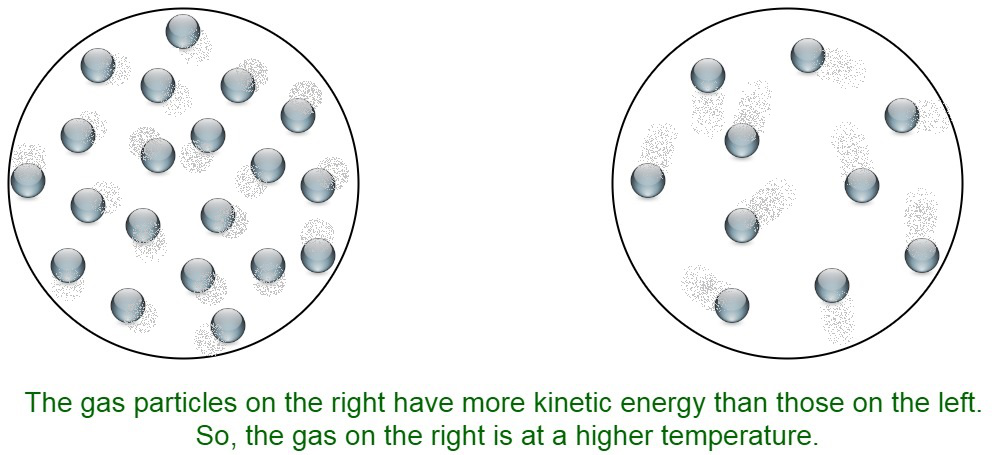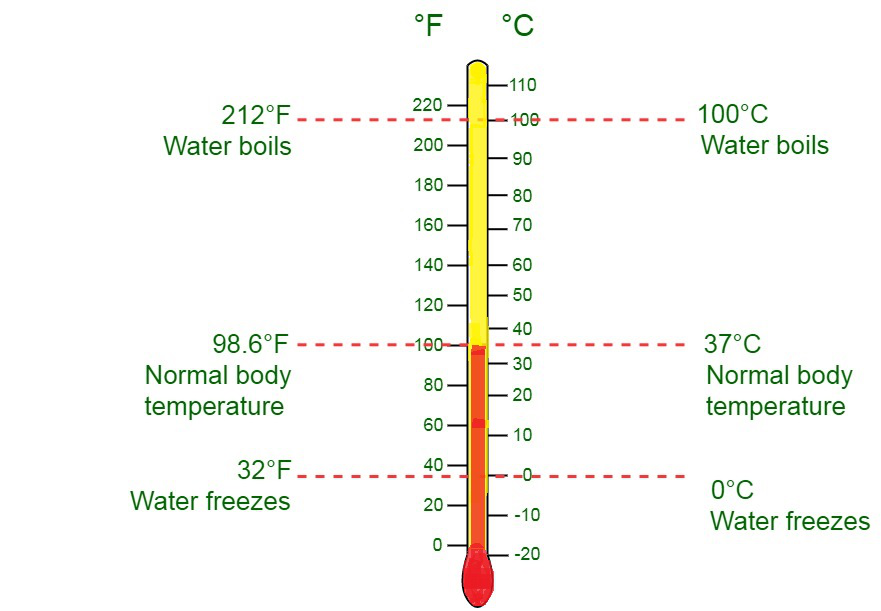Related Articles

# What is the Unit of Temperature?

• Last Updated : 14 Jul, 2021

Temperature is a measure of how hot or cold something is. It is a physical quantity. Temperature can be measured in terms of the kinetic energy of the particles. It defines the rate of movement of particles moving inside a material. It is considered to be an average measure. The higher the temperature, the faster is the movement of the particles composing the substance.

Temperature can be measured easily using a thermometer. Thermometers rely on a scale to measure the rate of hotness or coldness of a body. Upon heating the substance, the kinetic energy of the particles increases. As a result, the volume or mass of the substance changes. The expansion of the substance can be measured using the thermometer. The most widely used scale to measure temperature is Celsius. Temperature can be measured using degrees. It is calibrated by marking two fixed points on the body. The space between these two marked fixed points is broken up into a constant number of divisions termed degrees.Useful temperatures

When they invented temperature scales, scientists found there were certain things that were always around the same temperature:

• Water freezes at a temperature of 0 °C, 32 °F, or 273.15 K.
• Water boils at a temperature of 100o C or 373.15 K.
• The temperature inside the human body is generally observed to be 37 °C or 98 °F.

Absolute Zero

The coldest possible temperature to be observed is absolute zero. At absolute zero, the atoms and molecules come to rest position and have no heat energy to perform the movement. Absolute zero is assumed to be at a temperature of 0 K,-459 °F, or -273.15 °C. It is the lowest recorded absolute temperature. At this temperature, no energy can be removed from the matter or any substances in the form of any heat energy.

### S.I Unit of Temperature

The S.I unit of temperature is Kelvin, denoted by K. It was devised by Lord Kelvin. Though the scientists commonly use the scale Celsius or Centigrade (°C). Kelvin degree scale has the same measurement as the Celsius degree scale. In Kelvin’s scale, the zero point is 273.15 which is considered to be below that of the Celsius scale. Every division of the Kelvin scale is considered to be equivalent to a degree of the Celsius scale.

Kelvin scale is used in the cases where the temperature of substances where water is not involved is not measured. According to the Kelvin scale, the freezing point of water at 273.15oK, and the boiling point is observed to be at 373.15oK.

The Kelvin scale of temperature is also known as S.I. scale. It is recognized as the international standard for scientific temperature measurement.

Temperature in Kelvin = Temperature in oC + 273

Applications of Kelvin scale:

• It is used in lightning
• Scientific measurements
• Colour temperature determination### Common Unit of Temperature

The scale used in most of the world is in degrees Celsius, sometimes called “centigrade”. It is most widely used in everyday applications. It is a convenient and comfortable unit of temperature measurement since it measures the freezing point of water at 0o C and boiling points at 100o C respectively.

Celsius scale is also commonly known as the centigrade scale because of the 100-degree interval between the defined set of points. It can be used to measure both scientific temperatures as well as a temperature interval in between two observed points. It is characterized by two points namely, absolute zero and triple point.

Temperature in oC = Temperature in Kelvin – 273

Applications of Celsius scale:

• Metric system
• Scientific measurements

### Interconversion between units of temperature

The temperature units are easily inter-convertible to different formats by small formulae:

### Sample Questions

Question 1: Define the triple point of a substance.

The triple point of any substance can be termed as the unique temperature and pressure conditions at which all the three phases, that is, the solid, liquid and gas phases of a substance are all in equilibrium with each other.

Question 2: Why is Kelvin preferred over Celsius?

The Kelvin scale is preferred over Celsius scale because of the following two reasons :

• It is an absolute measure of temperature
• It is directly related to kinetic energy.
• It is directly related to the volume of the substance.

Question 3: Let us assume a temperature t to be recorded on both the Celsius and Fahrenheit scale. Determine the temperature t.

We have,

Converting between Celsius and Fahrenheit we have,

C/5 = F-32/9

Substituting temperature t,

=> t/5 = t-32/9

=> 9t = 5t – 160

=> 4t = -160

Therefore, the temperature = -40

Question 4: Why is absolute zero considered to be universal?